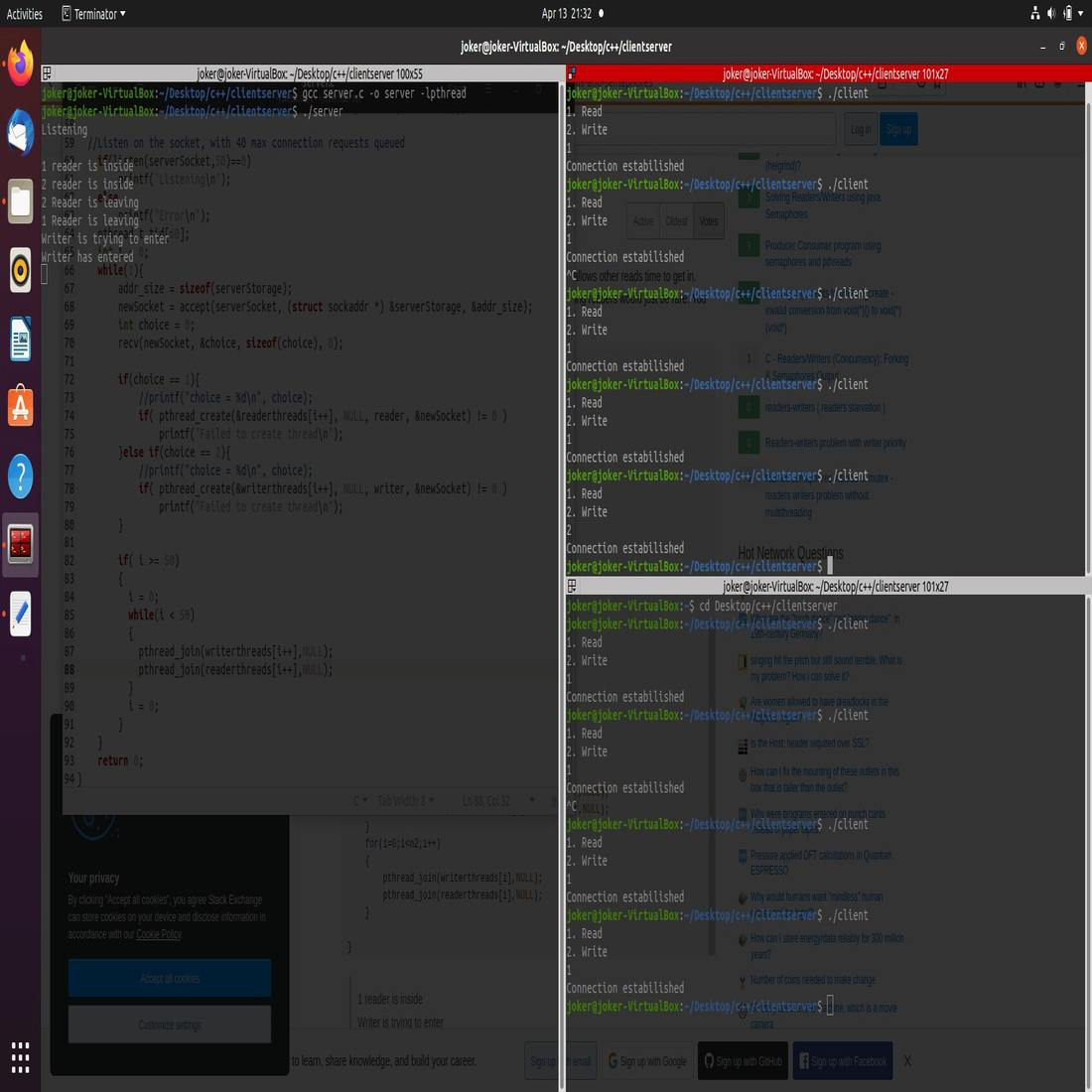Related Articles

# Handling multiple clients on server with multithreading using Socket Programming in C/C++

• Last Updated : 27 Aug, 2021

This tutorial assumes that the reader has a basic knowledge of socket programming, i.e has a familiarity with basic server and client models. In the basic model, the server handles only one client at a time, which is a big assumption if one wants to develop any scalable server model. The simple way to handle multiple clients would be to spawn a new thread for every new client connected to the server.

Semaphores: Semaphore is simply a variable that is non-negative and shared between threads. This variable is used to solve the critical section problem and to achieve process synchronization in the multiprocessing environment.

sem_post: sem_post() increments (unlocks) the semaphore pointed to by sem. If the semaphore’s value consequently becomes greater than zero, then another process or thread blocked in a sem_wait(3) call will be woken up and proceed to lock the semaphore.

```#include <semaphore.h>
int sem_post(sem_t *sem);```

sem_wait: sem_wait() decrements (locks) the semaphore pointed to by sem. If the semaphore’s value is greater than zero, then the decrement proceeds and the function returns, immediately. If the semaphore currently has the value zero, then the call blocks until either it becomes possible to perform the decrement (i.e., the semaphore value rises above zero), or a signal handler interrupts the call.

```#include <semaphore.h>
int sem_wait(sem_t *sem);```

Implementation: For the server-side, create two different threads; a reader thread, and a writer thread. First, declare a serverSocket, an integer, a variable to hold the return of socket function.

`int serverSocket = socket(domain, type, protocol);`
• serverSocket: Socket descriptor, an integer (like a file-handle).
• domain: Integer, communication domain e.g., AF_INET (IPv4 protocol), AF_INET6 (IPv6 protocol).
• type: Communication type.
• SOCK_STREAM: TCP(reliable, connection-oriented).
• SOCK_DGRAM: UDP(unreliable, connectionless).
• protocol: Protocol value for Internet Protocol(IP), which is 0. This is the same number that appears on the protocol field in the IP header of a packet.(man protocols for more details).

Then, after initializing all the necessary variables bind the socket.

bind: After the creation of the socket, the bind function binds the socket to the address and port number specified in addr(custom data structure). In the example code, we bind the server to the local host, hence INADDR_ANY is used to specify the IP address.

```int bind(int sockfd, const struct sockaddr *addr,

listen: It puts the server socket in a passive mode, where it waits for the client to approach the server to make a connection. The backlog defines the maximum length to which the queue of pending connections for sockfd may grow. If a connection request arrives when the queue is full, the client may receive an error with an indication of ECONNREFUSED.

`int listen(int sockfd, int backlog);`

Approach:

• After accepting the connection to the desired port, receive an integer from the client that defines the choice for reading or writing. Choice 1 indicates reader, while choice 2 indicates writer.
• After making successful connections to the server-client asks the user for input on the choice variable.
• After getting the choice from the user, the client then sends this choice to the server to call the reader or writer thread by creating a client thread for the request.

Below is the implementation of the above approach:

Code for Server Side:

## C

 `// C program for the Server Side` `// inet_addr``#include ` `// For threading, link with lpthread``#include ``#include ``#include ``#include ``#include ``#include ``#include ` `// Semaphore variables``sem_t x, y;``pthread_t tid;``pthread_t writerthreads;``pthread_t readerthreads;``int` `readercount = 0;` `// Reader Function``void``* reader(``void``* param)``{``    ``// Lock the semaphore``    ``sem_wait(&x);``    ``readercount++;` `    ``if` `(readercount == 1)``        ``sem_wait(&y);` `    ``// Unlock the semaphore``    ``sem_post(&x);` `    ``printf``(``"\n%d reader is inside"``,``           ``readercount);` `    ``sleep(5);` `    ``// Lock the semaphore``    ``sem_wait(&x);``    ``readercount--;` `    ``if` `(readercount == 0) {``        ``sem_post(&y);``    ``}` `    ``// Lock the semaphore``    ``sem_post(&x);` `    ``printf``(``"\n%d Reader is leaving"``,``           ``readercount + 1);``    ``pthread_exit(NULL);``}` `// Writer Function``void``* writer(``void``* param)``{``    ``printf``(``"\nWriter is trying to enter"``);` `    ``// Lock the semaphore``    ``sem_wait(&y);` `    ``printf``(``"\nWriter has entered"``);` `    ``// Unlock the semaphore``    ``sem_post(&y);` `    ``printf``(``"\nWriter is leaving"``);``    ``pthread_exit(NULL);``}` `// Driver Code``int` `main()``{``    ``// Initialize variables``    ``int` `serverSocket, newSocket;``    ``struct` `sockaddr_in serverAddr;``    ``struct` `sockaddr_storage serverStorage;` `    ``socklen_t addr_size;``    ``sem_init(&x, 0, 1);``    ``sem_init(&y, 0, 1);` `    ``serverSocket = socket(AF_INET, SOCK_STREAM, 0);``    ``serverAddr.sin_addr.s_addr = INADDR_ANY;``    ``serverAddr.sin_family = AF_INET;``    ``serverAddr.sin_port = htons(8989);` `    ``// Bind the socket to the``    ``// address and port number.``    ``bind(serverSocket,``         ``(``struct` `sockaddr*)&serverAddr,``         ``sizeof``(serverAddr));` `    ``// Listen on the socket,``    ``// with 40 max connection``    ``// requests queued``    ``if` `(listen(serverSocket, 50) == 0)``        ``printf``(``"Listening\n"``);``    ``else``        ``printf``(``"Error\n"``);` `    ``// Array for thread``    ``pthread_t tid;` `    ``int` `i = 0;` `    ``while` `(1) {``        ``addr_size = ``sizeof``(serverStorage);` `        ``// Extract the first``        ``// connection in the queue``        ``newSocket = accept(serverSocket,``                           ``(``struct` `sockaddr*)&serverStorage,``                           ``&addr_size);``        ``int` `choice = 0;``        ``recv(newSocket,``             ``&choice, ``sizeof``(choice), 0);` `        ``if` `(choice == 1) {``            ``// Creater readers thread``            ``if` `(pthread_create(&readerthreads[i++], NULL,``                               ``reader, &newSocket)``                ``!= 0)` `                ``// Error in creating thread``                ``printf``(``"Failed to create thread\n"``);``        ``}``        ``else` `if` `(choice == 2) {``            ``// Create writers thread``            ``if` `(pthread_create(&writerthreads[i++], NULL,``                               ``writer, &newSocket)``                ``!= 0)` `                ``// Error in creating thread``                ``printf``(``"Failed to create thread\n"``);``        ``}` `        ``if` `(i >= 50) {``            ``// Update i``            ``i = 0;` `            ``while` `(i < 50) {``                ``// Suspend execution of``                ``// the calling thread``                ``// until the target``                ``// thread terminates``                ``pthread_join(writerthreads[i++],``                             ``NULL);``                ``pthread_join(readerthreads[i++],``                             ``NULL);``            ``}` `            ``// Update i``            ``i = 0;``        ``}``    ``}` `    ``return` `0;``}`

Code for Client Side:

## C

 `// C program for the Client Side``#include ``#include ``#include ``#include ` `// inet_addr``#include ``#include ` `// For threading, link with lpthread``#include ``#include ` `// Function to send data to``// server socket.``void``* clienthread(``void``* args)``{` `    ``int` `client_request = *((``int``*)args);``    ``int` `network_socket;` `    ``// Create a stream socket``    ``network_socket = socket(AF_INET,``                            ``SOCK_STREAM, 0);` `    ``// Initialise port number and address``    ``struct` `sockaddr_in server_address;``    ``server_address.sin_family = AF_INET;``    ``server_address.sin_addr.s_addr = INADDR_ANY;``    ``server_address.sin_port = htons(8989);` `    ``// Initiate a socket connection``    ``int` `connection_status = connect(network_socket,``                                    ``(``struct` `sockaddr*)&server_address,``                                    ``sizeof``(server_address));` `    ``// Check for connection error``    ``if` `(connection_status < 0) {``        ``puts``(``"Error\n"``);``        ``return` `0;``    ``}` `    ``printf``(``"Connection estabilished\n"``);` `    ``// Send data to the socket``    ``send(network_socket, &client_request,``         ``sizeof``(client_request), 0);` `    ``// Close the connection``    ``close(network_socket);``    ``pthread_exit(NULL);` `    ``return` `0;``}` `// Driver Code``int` `main()``{``    ``printf``(``"1. Read\n"``);``    ``printf``(``"2. Write\n"``);` `    ``// Input``    ``int` `choice;``    ``scanf``(``"%d"``, &choice);``    ``pthread_t tid;` `    ``// Create connection``    ``// depending on the input``    ``switch` `(choice) {``    ``case` `1: {``        ``int` `client_request = 1;` `        ``// Create thread``        ``pthread_create(&tid, NULL,``                       ``clienthread,``                       ``&client_request);``        ``sleep(20);``        ``break``;``    ``}``    ``case` `2: {``        ``int` `client_request = 2;` `        ``// Create thread``        ``pthread_create(&tid, NULL,``                       ``clienthread,``                       ``&client_request);``        ``sleep(20);``        ``break``;``    ``}``    ``default``:``        ``printf``(``"Invalid Input\n"``);``        ``break``;``    ``}` `    ``// Suspend execution of``    ``// calling thread``    ``pthread_join(tid, NULL);``}`

Output:We provide nothing but the best curated videos and practice problems for our students. Check out the C Foundation Course and master the C language from basic to advanced level. Wait no more, start learning today!

My Personal Notes arrow_drop_up